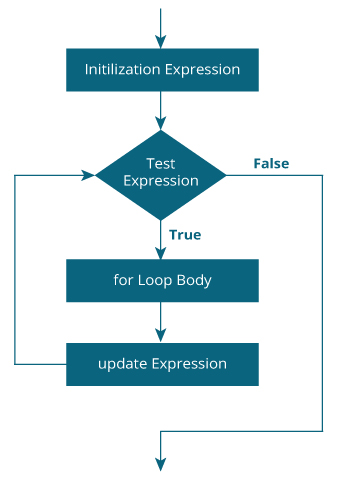### Php For loop

The for loop statement take three expressions inside its parenthesis. Separated by semi-colons.

The initialization expression is executed. This expression usually initializes one or more loop counter, but the syntax allows an expression of any degree of complexity.

The Condition expression is evaluated. If the value of condition is true, the loop statement execute. If the value of condition is false, the for loop terminates.

The update expression increment executes.

### Flow chart:### Result:

```The Number is 0
The Number is 1
The Number is 2
The Number is 3
The Number is 4
The Number is 5
The Number is 6
The Number is 7
The Number is 8
The Number is 9
The Number is 10
```